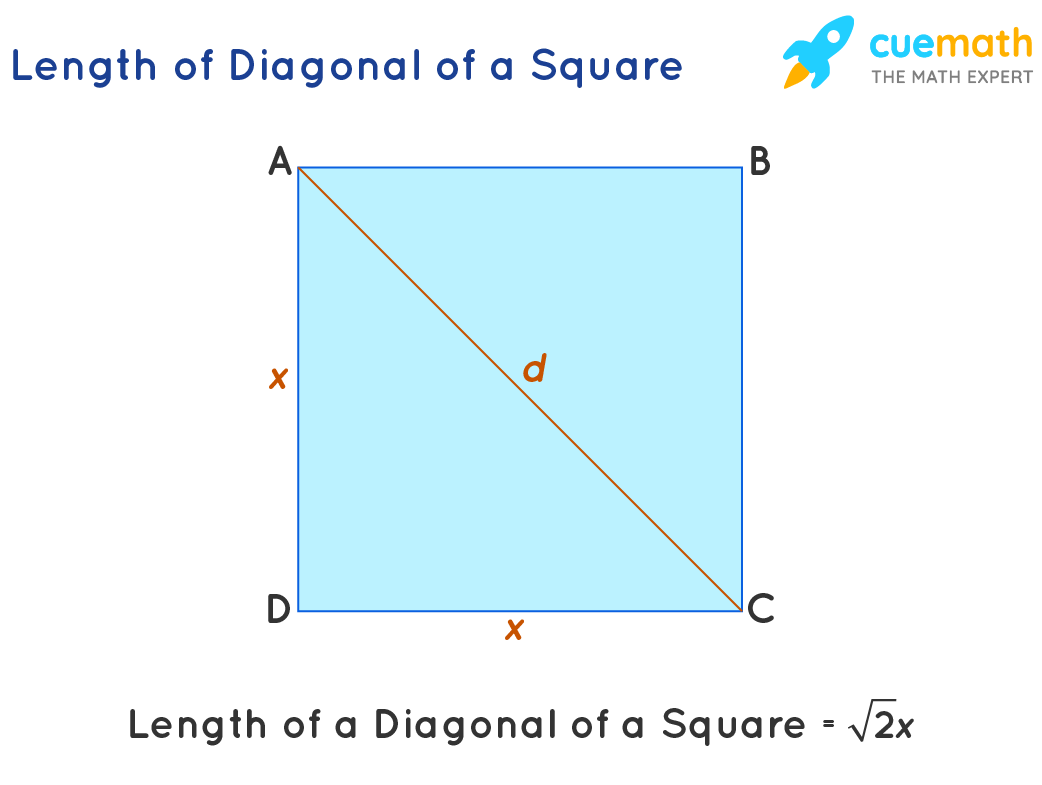# Diagonals Formula

Go back to  'Math Formulas'

A diagonal is a slant line or a sloping line that connects two vertices of a polygon, and those vertices should not be on the same edge. The word diagonal is derived from the Greek word “diagnosis” which means “from angle to angle”.  Let us discuss diagonals for different polygons such as square, rectangle, rhombus, parallelogram, etc. with its formulas.

## What is Formula for Diagonals?

The formula for Diagonals of a given polygon can be expressed as,

The number of diagonal lines of an n-sided polygon = n(n-3)/2
Square Diagonal = a√2
Rectangle Diagonal = √[l2 + b2]
Diagonal of a Rhombus, p = 2(A)/q and q = 2(A)/p
Parallelogram Diagonal
Diagonal, d1 = p = √[2x2+2y2 – q2]
Diagonal, d2 = q = √[2x2+2y2 – p2]Where
n is the number of sides of the polygon.
l is the length of the rectangle, b is the breadth of the rectangle.
p and q are diagonals of parallelogram and x,y are the sides of a parallelogram.
A is the area of the Rhombus

## Solved Examples Using Formula for Diagonals

### Example 1: Sam is walking around a rectangular park whose length of 10m and breadth is 8m. Find out the diagonal of a rectangular park where Sam is walking?

Solution:   To find: The diagonal of a rectangular park.

Given parameters are,

Length = 100m

Using Formula for Diagonals,

Rectangle Diagonal = √[l2 + b2]

= √[102 + 82 ]

= √

= 12.80 m2

### Example 2:The area of the rhombus is 100 inch2. Determine the second diagonal of a rhombus whose one of its diagonal measures is 10 inches.

Solution:  To find: The second diagonal of a rhombus

Given parameters are,

The area of the rhombus = 100 inch2

Using the Formula for Diagonals,

Diagonal of a Rhombus, p = 2(A)/q and q = 2(A)/p

p = 2(100)/10

p = 20 inch.